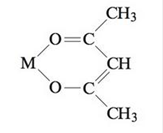Chapter 21, Problem 71AE

Chapter
Section
Textbook Problem

# Acetylacetone (see Exercise 43, part a), abbreviated acacH, is a bidentate ligand. It loses a proton and coordinates as acac−, as shown below:Acetylacetone reacts with an ethanol solution containing a salt of europium to give a compound that is 40.1% C and 4.71% H by mass. Combustion of 0.286 g of the compound gives 0.112 g Eu2O3. Assuming the compound contains only C, H, O, and Eu, determine the formula of the compound formed from the reaction of acetyl acetone and the europium salt. (Assume that the compound contains one europium ion.)

Interpretation Introduction

Interpretation: The formula of the compound formed from the reaction of acetylacetone and the europium salt using the given information.

Concept introduction: Acetylacetone abbreviated as acacH2 is a bidentate ligand. It loses a proton and coordinates as acac.

To determine: The formula of the compound formed from the reaction of acetylacetone and the europium salt.

Explanation

Explanation

Given

The mass of Eu2O3 is 0.112 g.

The mass percent of carbon is 40.1%.

The mass percent of hydrogen is 4.71%.

The molar mass of Eu2O3 is 351.96 g/mol.

The molar mass of Eu is 151.96 g/mol.

In 351.96 g of Eu2O3, the mass of Eu=303.92 g.

Therefore, in 0.112 g of Eu2O3, the mass of Eu=303.92351.96×0.112=0.0967 g

The mass percent of Eu is calculated by the formula,

MassofEuMassofcompound×100

The mass of compound is 0.286g.

The mass of Eu is 0.0967 g.

Substitute the value of mass of compound and mass of Eu in the above formula.

0.0967 g0.286g×100=33.8%Eu

The mass percent of oxygen is calculated by the formula,

Masspercentof O=100(MasspercentofEu+MasspercentofC+MasspercentofH)

The mass percent of Eu is 33.8%.

Substitute the value of mass percent in the above equation.

Masspercentof O=100(33.8+40.1%+4.71%)=21.39% O

The mass of the compound formed in the reaction is assumed to be 100 g.

The number of moles of Eu is calculated by the formula,

Moles of Eu=Mass of EuMolar mass of Eu

Mass of Eu is 33.8 g.

Substitute the value of mass and molar mass in the above equation.

Moles of Eu=33.8 g151.96 g/mol=0.222 mol Eu

The number of moles of C is calculated by the formula,

Moles of C=Mass of CMolar mass of C

Mass of C is 40.1 g.

Molar mass of C is 12.01 g/mol.

Substitute the value of mass and molar mass in the above equation.

Moles of C=40.1 g12.01 g/mol=3.34 mol C

The number of moles of H is calculated by the formula,

Moles of H=Mass of HMolar mass of H

Mass of H is 4

### Still sussing out bartleby?

Check out a sample textbook solution.

See a sample solution

#### The Solution to Your Study Problems

Bartleby provides explanations to thousands of textbook problems written by our experts, many with advanced degrees!

Get Started